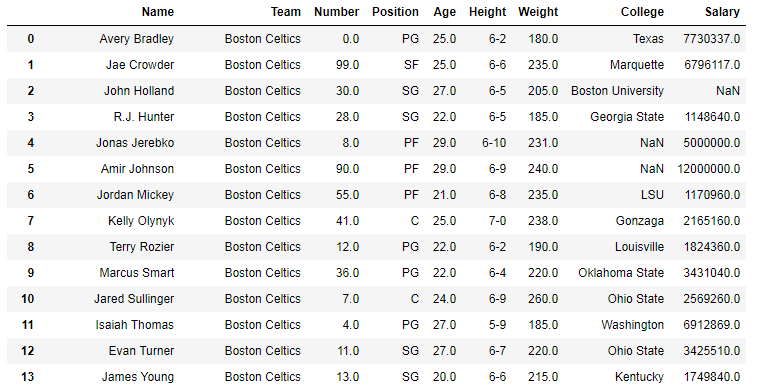Related Articles
Python | Pandas df.size, df.shape and df.ndim
• Last Updated : 03 Oct, 2018

Python is a great language for doing data analysis, primarily because of the fantastic ecosystem of data-centric Python packages. Pandas is one of those packages and makes importing and analyzing data much easier.

Pandas `.size`, `.shape` and `.ndim` are used to return size, shape and dimensions of data frames and series.

Syntax: dataframe.size
Return : Returns size of dataframe/series which is equivalent to total number of elements. That is rows x columns.

Syntax: dataframe.shape
Return : Returns tuple of shape (Rows, columns) of dataframe/series

Syntax: dataframe.ndim
Return : Returns dimension of dataframe/series. 1 for one dimension (series), 2 for two dimension (dataframe)

In the following examples, the data frame used contains data of some NBA players. The image of data frame before any operations is attached below.Example:
In this example, the output from size and shape is stored first. Since `.size` returns total number of elements, it is compared by multiplying rows and columns returned by the shape method. After that dimension of Dataframe and series is also checked using `.ndim`

 `# importing pandas module``import` `pandas as pd`` ` `# making data frame``data ``=` `pd.read_csv(``"https://media.geeksforgeeks.org/wp-content/uploads/nba.csv"``)`` ` `# dataframe.size``size ``=` `data.size`` ` `# dataframe.shape``shape ``=` `data.shape`` ` `# dataframe.ndim``df_ndim ``=` `data.ndim`` ` `# series.ndim``series_ndim ``=` `data[``"Salary"``].ndim`` ` `# printing size and shape``print``(``"Size = {}\nShape ={}\nShape x Shape = {}"``.``format``(size, shape, shape[``0``]``*``shape[``1``]))`` ` `# printing ndim``print``(``"ndim of dataframe = {}\nndim of series ={}"``.``format``(df_ndim, series_ndim))`

Output:

```Size = 4122
Shape=(458, 9)
Shape x Shape = 4122
ndim of dataframe = 2
ndim of series=1```

As it can be seen, rows x columns from .shape is equal to the value returned by .size
Also, ndim for dataframe was 2 and series is 1 which is true for all kind of dataframes and series.

Attention geek! Strengthen your foundations with the Python Programming Foundation Course and learn the basics.

To begin with, your interview preparations Enhance your Data Structures concepts with the Python DS Course. And to begin with your Machine Learning Journey, join the Machine Learning – Basic Level Course

My Personal Notes arrow_drop_up